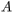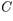# Problem: Six parallel-plate capacitors of identical plate separation have different plate areas ,different capacitances ,and different dielectrics filling the space between the plates.Part A. Rank the following capacitors on the basis of the dielectric constant of the material between the plates.Rank from largest to smallest.1. A = 4 cm2    C = 2 nF2. A = 1 cm2     C = 1 nF3. A = 2 cm2     C = 8 nF4. A = 8 cm2    C = 2 nF5. A = 4 cm2    C = 1 nF6. A = 2 cm2     C = 4 nF

###### FREE Expert Solution

Capacitance:

$\overline{){\mathbf{C}}{\mathbf{=}}\frac{\mathbf{k}{\mathbf{\epsilon }}_{\mathbf{0}}\mathbf{A}}{\mathbf{d}}}$

k is the dielectric constant, A is the area and d is the plate separation.

k = Cd/ε0

(1 m)2 = (100cm)2

k1 = (d/ε0) × (2 × 10-9)/(4 × 10-4) = (5.0 × 10-6)(d/ε0)

94% (449 ratings)###### Problem Details

Six parallel-plate capacitors of identical plate separation have different plate areas,different capacitances,and different dielectrics filling the space between the plates.

Part A. Rank the following capacitors on the basis of the dielectric constant of the material between the plates.

Rank from largest to smallest.

1. A = 4 cm2    C = 2 nF

2. A = 1 cm2     C = 1 nF

3. A = 2 cm2     C = 8 nF

4. A = 8 cm2    C = 2 nF

5. A = 4 cm2    C = 1 nF

6. A = 2 cm2     C = 4 nF